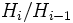# Finite characteristic series operator

This article defines a group property modifier (a unary group property operator) -- viz an operator that takes as input a group property and outputs a group property

## Definition

### Definition with symbols

The finite characteristic series operator is a group property modifier that takes as input a group property$p$ and outputs a group property$q$ such that a group$G$ satisfies$q$ if and only if it has a characteristic series$e = H_0 \le H_1 \le \ldots \le H_r = G$ such that each quotient$H_i/H_{i-1}$ satisfies property$p$.

## Properties

### Monotonicity

This group property modifier is monotone, viz if$p \le q$ are group properties and$f$ is the operator, then$f(p) \le f(q)$

### Ascendance

This group property modifier is ascendant, viz the image of any group property under this modifier is always weaker than the group property we started with

### Idempotence

This group property modifier is idempotent, viz applying it twice to a group property has the same effect as applying it once